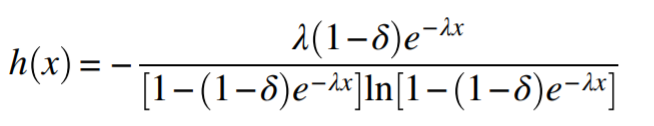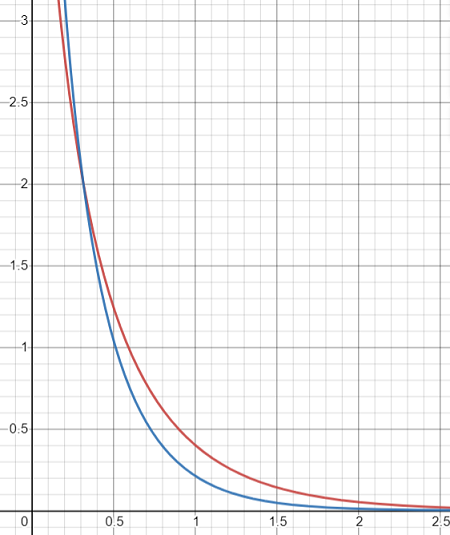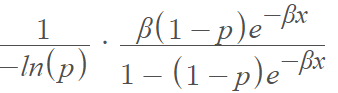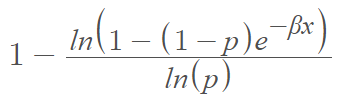# Exponential-Logarithmic Distribution

Share on

## What is the Exponential-Logarithmic Distribution?

The exponential-logarithmic (EL) distribution is a log-series mixture distribution of exponential random variables, introduced by Tahmasbi and Rezaei in 2008 . It is created when the rate parameter for the exponential distribution is randomized by the logarithmic distribution.

The distribution is used to model decreasing failure or survival rates; In other words, it can be used to model processes that improve over time. An example would be a trainee machine operator that makes fewer errors as training progresses. In reliability analysis, the distribution is based on the concept of modeling reliability when time-to-failure happens when an unknown number of initial “defects” on a components is considered . It is defined on the interval [0, ∞).

One way to define the distribution is by the functionSometimes you’ll see “p” instead of δ and β instead of λ.

## PDF & CDF of the Exponential-Logarithmic DistributionGraph of the PDF of the exponential-logarithm distribution, with p = 0.5 and β = 2 (red) and 3 (blue).

The probability density function (PDF) of the exponential-logarithm distribution is:The cumulative distribution function is:## References

Graph created with Desmos.
 Tahmasbi, R. and Rezaei, S. (2008). A two-parameter lifetime distribution with decreasing failure rate. Computational Statistics & Data Analysis 52(8):3889-3901
 Rahmouni, M. & Orabi, A. (2018). A Generalization of the Exponential-Logarithmic Distribution for Reliability and Life Data Analysis. Retrieved January 2, 2022 from:
https://arxiv.org/pdf/1803.01156.pdf
 King, M. Statistics for Process Control Engineers: A Practical Approach. (2017). Wiley.

CITE THIS AS:
Stephanie Glen. "Exponential-Logarithmic Distribution" From StatisticsHowTo.com: Elementary Statistics for the rest of us! https://www.statisticshowto.com/exponential-logarithmic-distribution/
---------------------------------------------------------------------------Need help with a homework or test question? With Chegg Study, you can get step-by-step solutions to your questions from an expert in the field. Your first 30 minutes with a Chegg tutor is free!